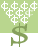Rags to Riches: Answer questions in a quest for fame and fortune.# Radical Expressions Can you win the MILLION Dollars?

Simplify each radical expression. If a variable expression is not positively positive after finding the square root then be sure the use absolute value bars on it. sqrt(x^2) is |x| while sqrt(x^4) is x^2 . Recall the ^ indicates an exponent.This activity was created by a Quia Web subscriber. Learn more about QuiaCreate your own activities